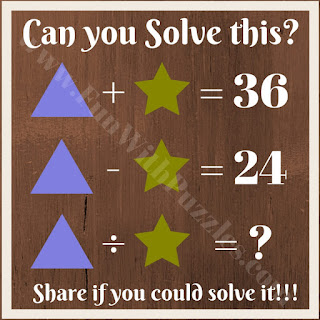As a kid, I used to love to solve math equations. In most of these math puzzles, alphabets are given in the equations and one has to find the value of these alphabets. However, these math equations become more fun when these alphabets are replaced with pictures. We have earlier published animal math picture puzzles as well as fruit math picture puzzles in which one has to calculate the value of a given animal or fruit. These math equation riddles are algebraic puzzles with shapes. These are all easy math puzzles to be solved by kids.
In these kids' math equation riddles, two equations are given which you have to solve for the two given triangular shapes.  Once you are able to find the value of each of these shapes and solve the third equation to get the required answer.
These math equations riddles are easy puzzles for kids.1. Easy math puzzle for kids2. Maths puzzle quiz for kids3. Maths brain teaser question for kids4. Kid's math equation game5. Maths puzzle riddle for kids

## Answers to Kid's Math Equations Riddles

Do checkout below listed Maths Puzzles and Brain Teasers to challenge your brain

## Matchstick Maths Picture Puzzles for Kids with Answers

It contains fun Maths Picture Puzzles for kids in which one has to solve the Maths equations by moving exactly one matchstick.

## Interesting Maths IQ Riddles for Kids and Teens With Answers

It has a mix of interesting Maths brain teasers which will test your brain IQ.

## Brain Teasers & Riddles IQ Test for Teens & Adults with answers

It contains another set of Brain Teasers and Riddles that are tricky and will tickle your brain.

## Simple Fun Brain Teasers for Kids with Answers

In the end, simple fun brain teasers are created by kids. These puzzles require a specific kids mindset to solve. So teens and adults will find these simple puzzles as tough.